# Numb3rs 418: When Worlds Collide

In this episode there was an unfortunately low quantity of mathematics, so we'll just use one thing that was mentioned as a starting point for discussing some interesting math.

In one of the "math view" sections Charlie is talking while a picture that has two possible interpretations is being shown. The picture below is an example because it can either look like two faces or like a vase. This effect is called the figureground effect. They subtly imply that this effect is partially caused by the angle at which you are looking at the picture. Based on personal experiments, the author of this page doesn't think this is true. However, it does lead into a discussion of rotations in 3 dimensions.

## The Orthogonal Group

Let's think about a rigid sphere in 3 dimensions. What kinds of ways can we move a sphere so that it looks the same after we move it? (We're assuming that it's completely symmetrical.) If it looks the same, it must be in the same place, so the only possible movements are rotations. Now if you do one rotation and then another one, if you consider the two movements as one movement, it's still a rotation. Also, for any rotation there is an "inverse" rotation, which will move the sphere back to its original position. Also, if you don't make any movement at all, the sphere still looks the same. All these facts mean that the set of all rotations form what is called a "group."

Groups have been studied implicitly for a very long time, since people have been studying integers for thousands of years. However, the concept wasn't completely formalized until around 1870. This involved the work of prominent mathematicians such as Galois (pictured), Cauchy, and Cayley, among many others. There has been a huge amount of work done to understand these simple axioms, and collecting all this together would probably take over 100,000 pages. However, there are still many unanswered questions about groups.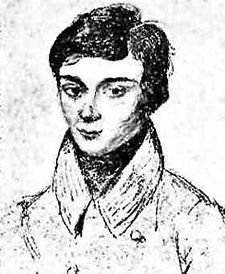For clarity, we'll define what it means to be a group more carefully. Let G be a set, and assume we have a function which we'll call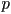that takes as input two elements of G and returns 1 element of G as an output. This function is called the group operation. If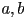are two elements of G, we'll writefor the element of G that is returned by. For G to be a group with operation p, we require p to have certain nice properties. The first is called associativity, which means that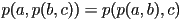. The second is that G must have an identity element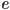, which means that for any element g in G, we have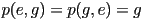. The final property is that any element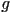must have an inverse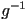, which means that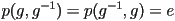. Now let's give a couple examples. First, let G be the set of integers, and if a,b are integers, let. One of the basic things we learn in elementary school is that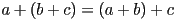, which means that addition satisfies the associativity condition that we required. Also, for any integer,, so 0 is the identity element. Finally, for any integer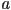, the negative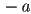is also an integer, and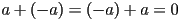, so each integer has an inverse under addition. Since we have verified that the integers under addition satisfy all our requirements, this means that they form a group.

Two Examples of a Group
1. Use the discussion in the above two paragraphs to verify that if we let G be the set of all possible rotations of the sphere and p be composition, (i.e. if a,b are two rotations, then p(a,b) is the rotation given by first rotating by a, and then rotating by b), then G is a group. This group is called SO(3).
2. Let G be the set of nonnegative real numbers and p(a,b) = a*b be multiplication. Show that G is a group with operation p.
3. Can you think of other simple examples of a group? (Hint: Think about rotations of rigid objects other than the sphere, or of matrix multiplication.)
The concept of a "fiber bundle" is a very important concept in mathematics, although the formal definition of a fiber bundle came about much later than the first formal definition of a group. In the discussion we were talking about a "circle bundle," which is a particular example of a "fiber bundle." Now let's talk about another example, this time of a "line segment bundle." There is an example of this object that you have had experience with in real life. Can you guess what it is? It's a rubber band. To think of it as a line segment bundle, lay one down on a table so that the bottom of the rubber band forms a circle on the table. Then over each point on the circle there is a "line segment." To pick a point on the rubber band, it is enough to pick a point on the circle, and then to pick a point on the line segment. In this example, the circle is analogous to the sphere in the example in the discussion. The line segment here is the analog of the circle.

Now let's study the group SO(3) of rotations of the 2-dimensional sphere in a bit more detail. The first thing to do is to figure out how to specify a rotation completely. Let's fix a special point (to be concrete, let's say it's the north pole) on a sphere and see what information we get by figuring out where a rotation sends it. First, let's assume that some particular rotation fixes the north pole, i.e. after we apply that rotation, the north pole is in the same place. How many rotations will do this? Well, if the north pole is fixed, then that means that the equator will be rotated onto itself. Now let's pick a point on the equator and suppose that the rotation also fixes that point. So we're assuming that both the north pole and a point on the equator are both fixed by this rotation. So what are the possiblities for this rotation? Can it move any points? Since we're assuming that the sphere is completely rigid, if the rotation fixes these two points, it must actually fix every point. (We won't give a formal mathematical justification of this, but it makes intuitive sense. Imagine taking a globe and holding your finger on one point on the equator to hold that point still. Then the globe won't rotate at all.) So we now know that if we assume that a rotation holds the north pole fixed, we can specify exactly what that rotation is by specifying where a point on the equator goes. So there is a "circle" of possible rotations if the north pole is fixed. Now given an arbitraty rotation, we can determine where it takes the north pole, and then in the same way, there is only "a circle" of possibilities for what the rotation can do. This description of the set of all possible rotations actually means that SO(3) is "a circle bundle over the sphere," which is a fancy way of saying that to completely determine what a rotation does, first we pick a point on the sphere (which is where the north pole goes), and then there is a "circle" of possible rotations, and picking one point on that circle completely determines the rotation.

Fun Activity Involving a Mobious Strip
Get a newspaper and tear out a strip about 2 feet long and 4 inches wide. Tape it together to make a Mobius strip, which is a loop with a 1/2 twist in it. Get a pair of scissors and cut the strip down the middle (lengthwise). What is the result? Now take this and cut it down the middle again. What do you get now? Was this a surprise? (If you did it right, you should have gotten two seperate loops which are looped around each other.)